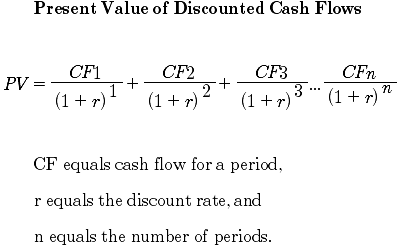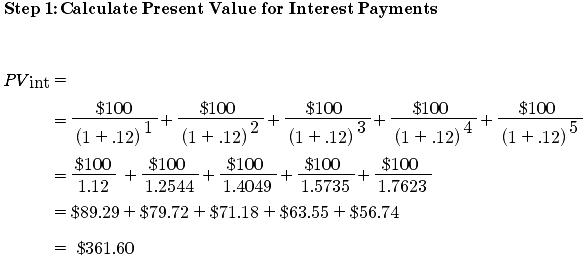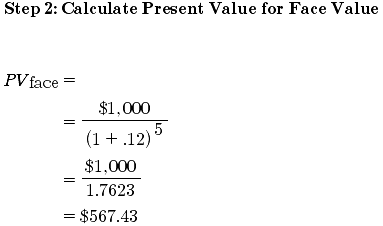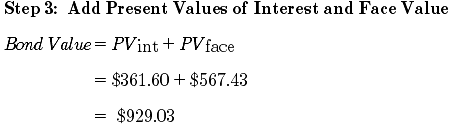# Bond Valuation: Formula, Steps & Examples

An error occurred trying to load this video.

Try refreshing the page, or contact customer support.

Coming up next: Determinants of Bond Yields

### You're on a roll. Keep up the good work!

Replay
Your next lesson will play in 10 seconds
• 0:04 Bond Terms
• 1:07 Discount Rates
• 2:51 Calculating the Bond Value
• 4:59 Corporate Investors
• 5:35 Selling the Bond…
• 6:29 Lesson Summary

Want to watch this again later?

Timeline
Autoplay
Autoplay
Speed

#### Recommended Lessons and Courses for You

Lesson Transcript
Instructor: James Walsh

M.B.A. Veteran Business and Economics teacher at a number of community colleges and in the for profit sector.

In this lesson, you'll learn the steps required to determine the value of a bond. You'll also learn how to come up with an appropriate discount rate to use in the bond value calculation.

## Bond Terms

Horse Rocket Software has issued a five-year bond with a face value of \$1,000 and a 10% coupon rate. Interest is paid annually. Similar bonds in the market have a discount rate of 12%. Before we calculate what this bond is worth, let's define some things. We'll first explain the term, give a definition, and then give a brief example of how the term is expressed in our example we opened with.

Term Definition Our Example
Face value The value of the bond to be paid at maturity \$1,000
Coupon rate The amount of interest to be received annually 10%
Discount rate The required return rate for an investor 12%
Maturity date The date the bond will be paid to the investor Five-year time period from the date of the purchase

## Discount Rate

The discount rate of bond valuation is subjective for each investor. It reflects the investor's evaluation of the entity issuing the bond in terms of how likely default might be. For example, a U.S. Treasury security will have a very low discount rate since the U.S. has never defaulted on its debts. A new business, on the other hand, will have a higher discount rate since the chances the business fails and defaults on its debts is much higher. A good starting point is the market rate for bonds of similar quality and risk.

A quick review of the amount, rates, and date from our opening example can help us out here. Why would an investor purchase a bond that pays 10% of the face value in annual interest payments when the discount rate is 12%? Ordinarily, they wouldn't, unless the company issuing the bond discounts the purchase price and offers the bond for less than the face value.

The bond is issued at a premium when the coupon rate is greater than the discount rate. The bond's purchase price is greater than the face value. An investor is willing to pay more than the face value because the expected cash flow from the bond will be greater than the required rate of return.

The bond is discounted when the coupon rate is less than the discount rate. The bond's purchase price is less than the face value. Because the expected cash flow from the bond is below the required rate of return, the investor will only purchase the bond when the price is below the face value. The Horse Rocket bond from our opening example will need to be discounted since the discount or required rate is greater than the coupon rate. The value of the bond will tell us how much the discount needs to be.

## Calculating the Bond Value

Calculating the value of a bond is a three-step process. Bonds have two income pieces. One is a stream of periodic interest payments the investor receives. The other is the principal repayment of the investment, which is made when the bond matures. What a bond is worth today is the combined present value of both of these two income pieces.

We begin by calculating the interest payments. Interest payments are paid quarterly, semi-annually, or annually for the duration of the bond. To keep things simple, the Horse Rocket bonds pay interest once per year. Our face value is, as your recall, \$1,000. And our coupon rate is 10%. That means we multiply \$1,000 by 0.10 and this produces our answer. We make an interest payment of \$100. Simple, right?

Next, we calculate the present values for the expected interest payments at a 12% discount rate for five years. Then we calculate the present value of the principal repayment. Here is the basic formula for finding the present value of a stream of cash flow:As we can see based on these calculations, to get the investor the desired return of 12% to compensate for the default risk these bonds carry, they will be willing to pay \$929.03.

To unlock this lesson you must be a Study.com Member.

### Register to view this lesson

Are you a student or a teacher?

### Unlock Your Education

#### See for yourself why 30 million people use Study.com

##### Become a Study.com member and start learning now.
Back
What teachers are saying about Study.com

### Earning College Credit

Did you know… We have over 160 college courses that prepare you to earn credit by exam that is accepted by over 1,500 colleges and universities. You can test out of the first two years of college and save thousands off your degree. Anyone can earn credit-by-exam regardless of age or education level.